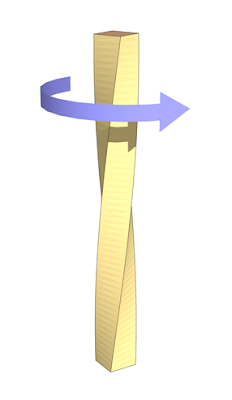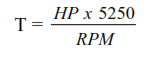# DEFINITION AND CALCULATIONS OF DRILL PIPE TORSIONAL STRENGTH (TORQUE)

## Drill Pipes TorqueThe torsional strength of Drill Pipe becomes critical when drilling deviated or horizontal holes or when pipe is stuck

The actual torque applied to the drill pipe during drilling is difficult to measure, but may be approximated by the following equation,where,
T       = torque delivered to DP, ft-lb
HP   = horse power used to produce rotation of pipe, hp
RPM = revolutions per minute

• If a tensile load is exerted on the drill pipe, the torsional strength values from Drill Pipes Handbook must be derated.

• al yield strength under tension may be calculated from the following equation,